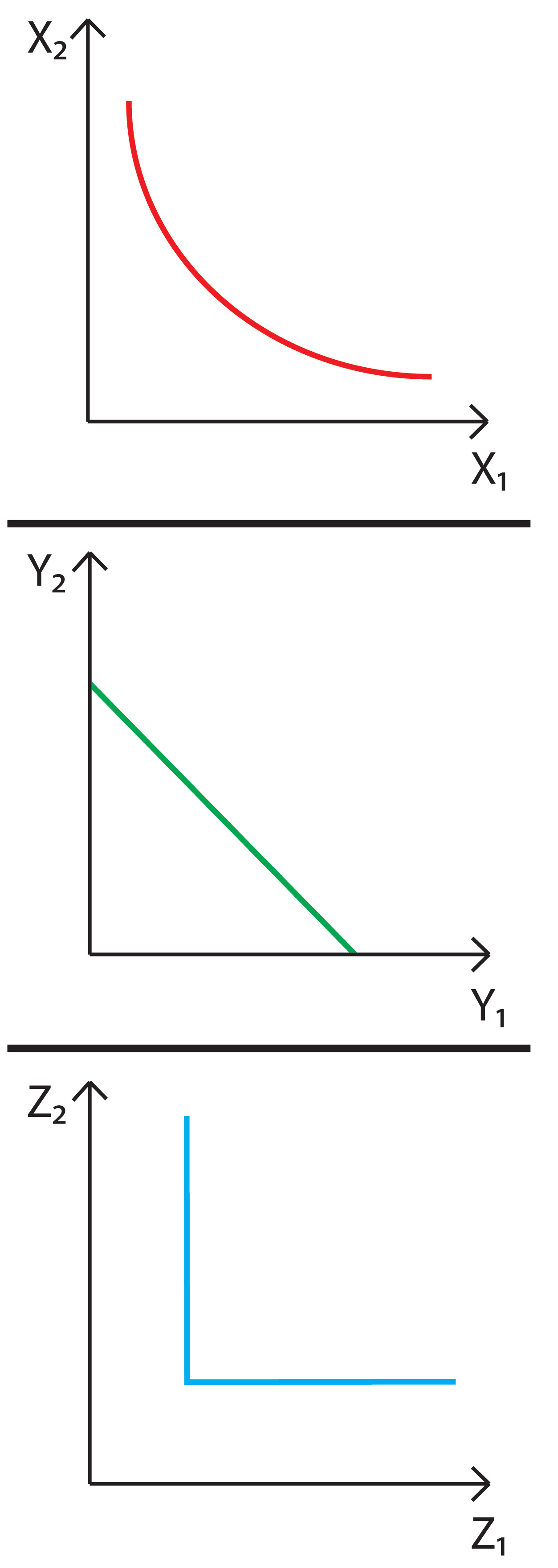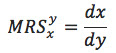### Ticker

6/recent/ticker-posts

# marginal rate of substitution (MRS)

The marginal rate of substitution (MRS) can be defined as how many units of good x have to be given up in order to gain an extra unit of good y, while keeping the same level of utility. Therefore, it involves the trade-offs of goods, in order to change the allocation of bundles of goods while maintaining the same level of satisfaction. It can be determined using the following formula:The MRS is linked with indifference curves, since the slope of this curve is the MRS. In the adjacent figure you can see three of the most common kinds of indifference curves.
The first one, which is generally used for defining the utility of consumption for a given economic agent, has a MRS that changes along the curve, and will tend to zero when diminishing the quantity of X2 and to infinite when diminishing the quantity of X1.
In the second graph, both goods are perfect substitutes, since the lines are parallel and the MRS = 1, that is the slope has an angle of 45º with each axis. When considering different substitutes goods, the slope will be different and the MRS can be defined as a fraction, such as 1/2 ,1/3, and so on. For perfect substitutes, the MRS will remain constant.
Lastly, the third graph represents complementary goods. In this case the horizontal fragment of each indifference curve has a MRS = 0 and the vertical fractions a MRS = ∞.